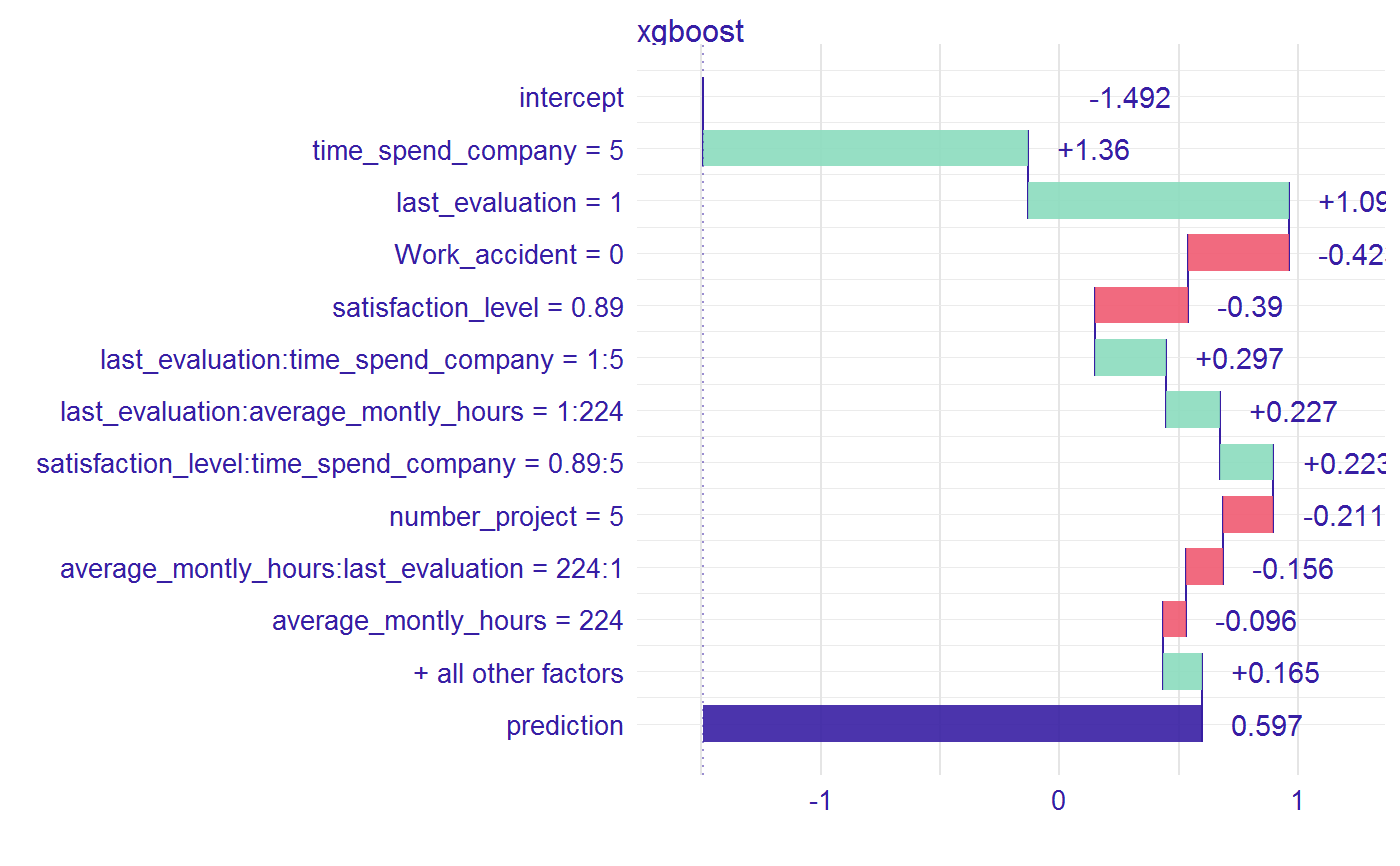This function calculates a table with influence of variables and interactions on the prediction of a given observation. It supports only xgboost models.

waterfall(xgb_model, new_observation, data, type = "binary",
option = "interactions", baseline = 0)

## Arguments

xgb_model a xgboost model. a new observation. row from the original dataset with the new observation to explain (not one-hot-encoded). The param above has to be set to merge categorical features. If you dont wont to merge categorical features, set this parameter the same as new_observation. the learning task of the model. Available tasks: "binary" for binary classification or "regression" for linear regression. if "variables", the plot includes only single variables, if "interactions", then only interactions. Default "interaction". a number or a character "Intercept" (for model intercept). The baseline for the plot, where the rectangles should start. Default 0.

## Value

an object of the broken class

## Details

The function contains code or pieces of code from breakDown code created by Przemysław Biecek and xgboostExplainer code created by David Foster.

## Examples


library("EIX")
library("Matrix")
sm <- sparse.model.matrix(left ~ . - 1,  data = HR_data)

library("xgboost")
param <- list(objective = "binary:logistic", max_depth = 2)
xgb_model <- xgboost(sm, params = param, label = HR_data[, left] == 1, nrounds = 25, verbose=0)

data <- HR_data[9,-7]
new_observation <- sm[9,]

wf <- waterfall(xgb_model, new_observation, data,  option = "interactions")
wf#>                                                         contribution
#> xgboost: intercept                                            -1.492
#> xgboost: time_spend_company = 5                                1.360
#> xgboost: last_evaluation = 1                                   1.093
#> xgboost: Work_accident = 0                                    -0.423
#> xgboost: satisfaction_level = 0.89                            -0.390
#> xgboost: last_evaluation:time_spend_company = 1:5              0.297
#> xgboost: last_evaluation:average_montly_hours = 1:224          0.227
#> xgboost: satisfaction_level:time_spend_company = 0.89:5        0.223
#> xgboost: number_project = 5                                   -0.211
#> xgboost: average_montly_hours:last_evaluation = 224:1         -0.156
#> xgboost: average_montly_hours = 224                           -0.096
#> xgboost: time_spend_company:last_evaluation = 5:1              0.095
#> xgboost: salary = 2                                            0.074
#> xgboost: satisfaction_level:number_project = 0.89:5           -0.003
#> xgboost: prediction                                            0.597
plot(wf)